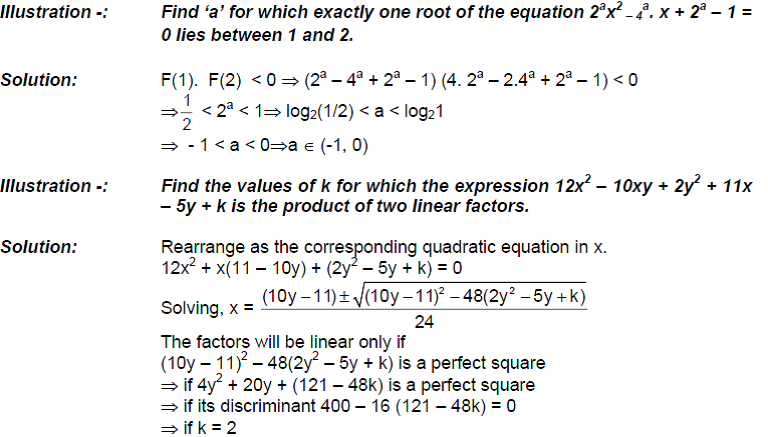ROOTS LIE IN AN INTERVAL

Here basically we will discuss different necessary and sufficient conditions we should impose on a quadratic equation ax2 + bx + c = 0 such that roots of the given equation lies in aIllustrations on Roots in an Interval

Illustration -: For the quadratic equation x2 – (m – 3)x + m = 0, find the value of m for which

(i)        one root is smaller than 2 and the other is greater than 2

(ii)       both roots are grater than 2

(iii)      both roots lie in (1, 2)

(iv)      exactly one root lie in (1, 2)